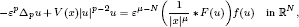Home

# Investigating the multiplicity and concentration behaviour of solutions for a quasi-linear Choquard equation via the penalization method

## Abstract

We study the multiplicity and concentration behaviour of positive solutions for a quasi-linear Choquard equationwhere Δ p is the p-Laplacian operator, 1 < p < N, V is a continuous real function on ℝ N , 0 < μ < N, F(s) is the primitive function of f(s), ε is a positive parameter and * represents the convolution between two functions. The question of the existence of semiclassical solutions for the semilinear case p = 2 has recently been posed by Ambrosetti and Malchiodi. We suppose that the potential satisfies the condition introduced by del Pino and Felmer, i.e.V has a local minimum. We prove the existence, multiplicity and concentration of solutions for the equation by the penalization method and Lyusternik–Schnirelmann theory and even show novel results for the semilinear case p = 2.

# Investigating the multiplicity and concentration behaviour of solutions for a quasi-linear Choquard equation via the penalization method

## Metrics

### Full text viewsFull text views reflects the number of PDF downloads, PDFs sent to Google Drive, Dropbox and Kindle and HTML full text views.

Total number of HTML views: 0
Total number of PDF views: 0 *Loading metrics...

### Abstract viewsAbstract views reflect the number of visits to the article landing page.

Total abstract views: 0 *Loading metrics...

* Views captured on Cambridge Core between <date>. This data will be updated every 24 hours.

Usage data cannot currently be displayed.